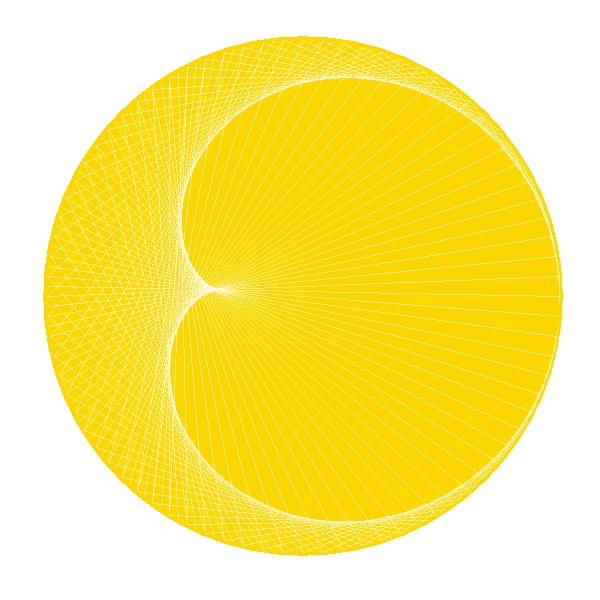# The Moon And The Sun

Do not swear by the moon, for she changes constantly. Then your love would also change (William Shakespeare, Romeo and Juliet)

The sun is a big point ant the moon is a cardioid:Here you have the code. It is a simple example of how to use `ggplot`:

```library(ggplot2)
n=160
t1=1:n
t0=seq(from=3, to=2*n+1, by=2) %% n
t2=t0+(t0==0)*n
df=data.frame(x1=cos((t1-1)*2*pi/n), y1=sin((t1-1)*2*pi/n), x2=cos((t2-1)*2*pi/n), y2=sin((t2-1)*2*pi/n))
opt=theme(legend.position="none",
panel.background = element_rect(fill="white"),
panel.grid = element_blank(),
axis.ticks=element_blank(),
axis.title=element_blank(),
axis.text =element_blank())
ggplot(df, aes(x = x1, y = y1, xend = x2, yend = y2)) +
geom_point(x=0, y=0, size=245, color="gold")+
geom_segment(color="white", alpha=.5)+opt
```